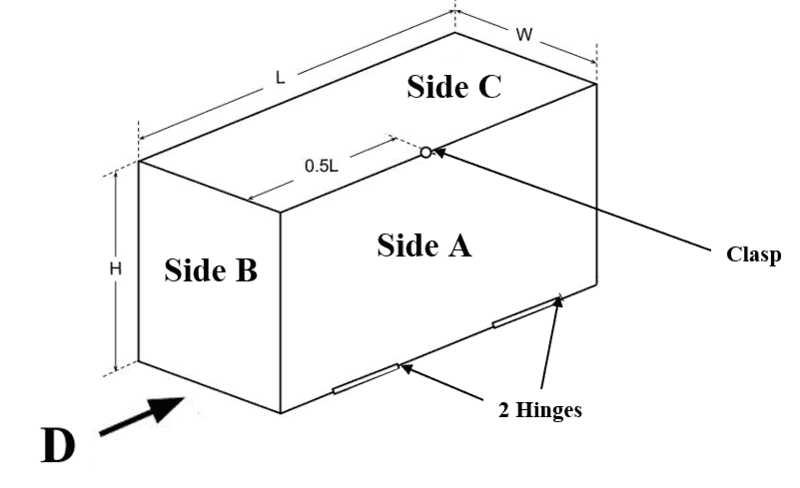# Hinged Water Storage Tank -- Calculate the force on one side

Gregs6799

## Homework Statement

A hinged water storage is shown below. Its top side is denoted as C, the narrow side as B and the long side as A. Assume water density is 1000 kg/m3 and gravity is 9.81 m/s2
Side A is hinged at the bottom edge and secured using a clasp at its top edge.
Dimensions are: L = 1m, H = 0.5m & W = 0.5m

Calculate the size and location of the water thrust force on side A

2. Homework Equations

I = bh^3 / 12

## The Attempt at a Solution

[/B]
I've got the correct 1st moment but not the 2nd moment
I submitted this:
2nd moment of area
I = bh^3 / 12
I = 1 x 0.5^3 / 12 = 0.0104
1.00 x 0.5^2 / 12 + 1.00 x 0.5 x 0.25^2 = 0.0520 and been told the formula is wrong but in my notes it says I = bh^3 / 12 is the correct formula.

Any help would be really appreciated.
Greg.

Last edited by a moderator:

Mentor
Would it be possible to provide the diagram?

Gregs6799(a)

L = 1m
H = 0.5m
W = 0.5m
Area = 1 x 0.5 = 0.5
Y = 0.5 / 2 = 0.25
1st moment of area = 0.5 x 0.25 = 0.125
Water thrust force
F = p x g x 1st moment of area
F = 1000 x 9.81 x 0.125 = 1,226.25N

My correct answer for 1st moment.

#### Attachments

Homework Helper
I believe that I see the problem. The hinge is on the bottom. If it were on the top your answer would be correct.

This can be understood as an integration. For the force calculation, you are integrating pressure at depth h times length of a horizontal strip as the depth goes from zero (at the top surface) to H (at the bottom). For the torque calculation, you should be integrating pressure at depth h times length of a horizontal strip times distance from the hinge as the depth goes from zero (at the top surface) to H (at the bottom).

Last edited:
Mentor
I think it's a really bad idea to memorize formulas. The much preferable objective should be to learn the fundamentals, and practice applying these fundaments. The more fundamental equation to begin with is $$p=\rho g z$$ where z is the depth measured downward from the top of the box. So the differential force acting on the section of door between depth z and depth z + dz is $$dF=pLdz=\rho g Lz dz$$ The moment of this force about the bottom hinge is $$dM=(H-z)dF=\rho g L(H-z)z dz$$
From these equations, what do you get for the total force F and the total moment M?

•jbriggs444
Gregs6799
I've got:
H = 0.5
z = 0.5
d = 0.5
F = 1000

H - z = (0.5 - 0.5) = 0,
d x F = 0.5 x 1000 = 500
z x d x z = (0.5 - 0.5) = 0, 0.5 x 0.5 x 0.5 = 0.125m
Which is my 1st moment for the 2nd moment I've been told should be about the hinge so bh^3/ 3 = 0.0416 (not bh^3/12)
0.0416 / 0.125 = 0.3328m

No doubt gone very wrong somewhere though.

Mentor
I've got:
H = 0.5
z = 0.5
d = 0.5
F = 1000

H - z = (0.5 - 0.5) = 0,
d x F = 0.5 x 1000 = 500
z x d x z = (0.5 - 0.5) = 0, 0.5 x 0.5 x 0.5 = 0.125m
Which is my 1st moment for the 2nd moment I've been told should be about the hinge so bh^3/ 3 = 0.0416 (not bh^3/12)
0.0416 / 0.125 = 0.3328m

No doubt gone very wrong somewhere though.
z is the integration variable, not a constant. You are supposed to integrate with respect to z from 0 to H, not just set z equal to the value of the depth at the bottom. Have you not learned how to do integration yet?

Gregs6799
My notes don't cover integration.

Mentor
My notes don't cover integration.

Gregs6799
No calculus either I'm afraid the notes are pretty much physics and very little math.
But is bh^3 / 3 not along the right path? And something like:
1.00 x 0.5^2 / 3 + 1.00 x 0.5 x 025^2 = 0.1145m
0.1145 / 0.125 = 0.916m

Mentor
No calculus either I'm afraid the notes are pretty much physics and very little math.
But is bh^3 / 3 not along the right path? And something like:
1.00 x 0.5^2 / 3 + 1.00 x 0.5 x 025^2 = 0.1145m
0.1145 / 0.125 = 0.916m
Are you saying you haven't had calculus, or are you saying that the course is not supposed to involve calculus? Or both?

Gregs6799
Yes both

Homework Helper
Without calculus, there is an approach that I believe could be made to work.

1. Pretend the hinge is at the top. Compute the torque by using the second moment of area.
2. Move the hinge to the bottom using the parallel axis theorem to compute the change in torque due to the change in axis.

Gregs6799
I'll have a look at that tomorrow, I've at work soon, thanks!

Gregs6799
So pretending the hinge is at the top I've got:
Iy (instead of Ix) = 1^3 x 0.5 / 3 = 0.166m
Iy(0.166) + Ad^2 (0.5 x 0.5^2) = 0.291m